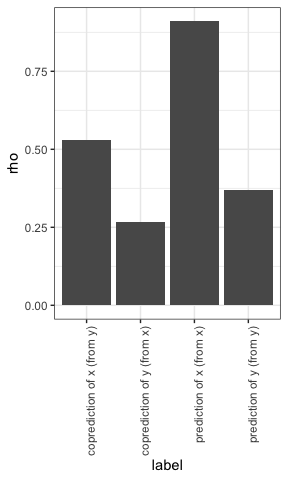# Introduction

The goal of co-prediction is to quantify dynamic similarity between two time series. Given two time series, $$x$$ and $$y$$, we assume that the dynamics can be represented as: $x_{t+tp} = F\left(\vec{x}_t\right) = F\left(\langle x_t, x_{t-\tau}, \dots, x_{t-(E-1)\tau} \rangle \right)$ and $y_{t+tp} = G\left(\vec{y}_t\right) = G\left(\langle y_t, y_{t-\tau}, \dots, y_{t-(E-1)\tau} \rangle \right)$ . Then co-prediction is a way to quantify how closely $$F$$ and $$G$$ resemble each other.

# Method

We can accomplish this task using rEDM by constructing concatenated time series and applying simplex or s-map to make predictions with appropriately defined libs and preds.

First, we grab some demo time series from the block_3sp data.frame:

library(rEDM)
data(block_3sp)

x <- block_3sp$x_t y <- block_3sp$y_t

Concatenate the time series and record which portions correspond to x and y:

concatenated_xy <- c(x, y)
lib_x <- c(1, length(x))
lib_y <- length(x) + c(1, length(y))

Use simplex to identify the optimal embedding dimension for x and use it to co-predict from x to y:

simplex_out_x <- simplex(concatenated_xy, lib = lib_x, pred = lib_x)
## Warning in model$run(): Found overlap between lib and pred. Enabling cross- ## validation with exclusion radius = 0. best_E_x <- simplex_out_x$E[which.max(simplex_out_x$rho)] copred_x_to_y <- simplex(concatenated_xy, lib = lib_x, pred = lib_y, E = best_E_x) and in the reverse direction: simplex_out_y <- simplex(concatenated_xy, lib = lib_y, pred = lib_y) ## Warning in model$run(): Found overlap between lib and pred. Enabling cross-
## validation with exclusion radius = 0.
best_E_y <- simplex_out_y$E[which.max(simplex_out_y$rho)]

copred_y_to_x <- simplex(concatenated_xy, lib = lib_y, pred = lib_x, E = best_E_y)

# Results

We can interpret the strength of dynamic similarity in comparison to the univariate predictability of x and y.

First, merge the output into a single data.frame:

to_plot <- data.frame(label = c("prediction of x (from x)",
"coprediction of x (from y)",
"prediction of y (from y)",
"coprediction of y (from x)"),
rbind(simplex_out_x[which.max(simplex_out_x$rho), ], copred_y_to_x, simplex_out_y[which.max(simplex_out_y$rho), ],
copred_x_to_y)
)

Plot the output

library(ggplot2)
ggplot(to_plot, aes(x = label, y = rho)) +
geom_col() + theme_bw() +
theme(axis.text.x = element_text(angle = 90, hjust = 1))